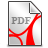# the RH, the 3D-NSE and the YME millennium problems

Disclaimer: all papers of the sections A-C are without authorization from the ivory tower

for latest updates and related papers see

www.riemann-hypothesis.de

www.unified-field-theory.de

www.euler-mascheroni-constant.de

A. A Kummer function based Zeta function theory

to enable proofs of

the Riemann Hypothesis and the Goldbach conjectureThe Riemann Zeta functionBraun K., A toolbox to solve the RH and to build a non-harmonic Fourier series based two-semicircle method.pdf (868.27KB)Braun K., A toolbox to solve the RH and to build a non-harmonic Fourier series based two-semicircle method.pdf (868.27KB)

B. A global unique H(1/2) (potential energy) inner product based weak solution of the 3D-Navier-Stokes equations

We provide a global unique (weak, generalized Hopf) H(1/2)-solution of the generalized 3D Navier-Stokes initial value problem. The global boundedness of a generalized energy inequality with respect to the energy Hilbert space H(1/2) is a consequence of the Sobolevskii estimate of the non-linear term (1959). The extended (energy) Hilbert space is in line with the proposed quantum potential energy space as proposed in section C. It provides a mathematical model for Mie's theory accompanied by Mie’s concept of an electric pressure enhancing the Maxwell equations. Mie's concept can be applied to the second unknown function in the NSE, the pressure p;  the pressure function p can be represented as Riesz operator transforms of (u x u), while the gradient (force) operator applied to the unknown pressure function p becomes the Calderón-Zygmund integrodifferential operator applied to the (velocity) NSE-solution function u (EsG) p. 44.Braun K., Global existence and uniqueness of 3D Navier-Stokes equations_1.pdf (483.9KB)Braun K., Global existence and uniqueness of 3D Navier-Stokes equations_1.pdf (483.9KB)

For more details concerning the H(1/2) "potential energy" inner product we refer to the following section C. Further supporting papers areJ. A. Nitsche footprints related to NSE problems.pdf (794.84KB)J. A. Nitsche footprints related to NSE problems.pdf (794.84KB)Braun K., The Prandtl (hypersingular) integral equation with double layer potential and the exterior Neumann problem.pdf (315.97KB)Braun K., The Prandtl (hypersingular) integral equation with double layer potential and the exterior Neumann problem.pdf (315.97KB)Braun K., Unusual Hilbert and Hoelder space frames for the (elementary particles) transport (Vlasov) equation.pdf (1.11MB)Braun K., Unusual Hilbert and Hoelder space frames for the (elementary particles) transport (Vlasov) equation.pdf (1.11MB)Braun K., Generalized wavelet theory and non-linear, non-periodic boundary value problems.pdf (439.35KB)Braun K., Generalized wavelet theory and non-linear, non-periodic boundary value problems.pdf (439.35KB)Braun K., Some remarkable pseudo-differential operators of order -1, 0, 1.pdf (664.7KB)Braun K., Some remarkable pseudo-differential operators of order -1, 0, 1.pdf (664.7KB)Braun K., A new ground state energy model.pdf (1.07MB)Braun K., A new ground state energy model.pdf (1.07MB)

C. A Krein space based quantum potential energy theory & a related model for the non-linear Landau damping phenomenon

www.unified-field-theory.deThe Bose-Einstein condensate

Abstract

A Krein space based matter field theory is provided. From the Mie theory the concept of discrete energy knots is taken modelled by a physical problem specific (self-adjoint) kinetic energy operator. From the correspondingly defined Krein space framework the concept of a (self-adjoint) potential energy operator is applied. It enables the definition of potential energy norms on all of the Krein space built on sets of quantum numbers leading to a (vacuum, plasma, Mie) grouping of the concerned quantum elements. The proposed model provides an appropriate framework for the Mie theory, an enhanced Maxwell theory accompanied by the concept of an electric pressure. This Mie theory makes the YME and its underlying mass gap problem obsolete.Braun, K., A Krein space based quantum potential theory.pdf (900.83KB)Braun, K., A Krein space based quantum potential theory.pdf (900.83KB)

D. PhD thesisJune 1986, Interior error estimates of the Ritz method for Pseudo-Differential equations (3).pdf (2.44MB)June 1986, Interior error estimates of the Ritz method for Pseudo-Differential equations (3).pdf (2.44MB)

The paper includes a proof of the quasi-optimal approximation behavior of the Ritz-Galerkin method in a Hilbert scale framework. The example 2 gives the model operator of the Symm (Pseudo-Differential) integral operator. Other examples would be the Calderon-Zygmund (singular integral) operator or the Hilbert transform (singular integral) operator.